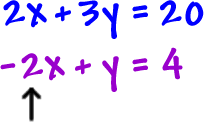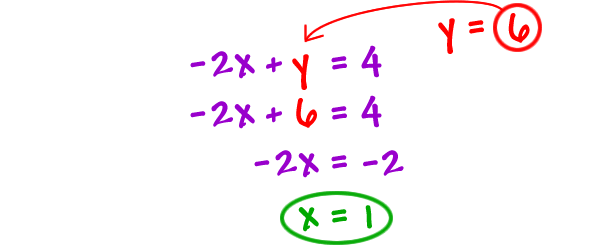Let's just do one and you'll see how it works:See how these guys are the same,
but with a different sign?

If we add the two equations -- straight down, those x critters are going to drop right out!

Just add "like terms" and drag the "=" down to:We've got one of them...  Now, we just need to get the x.  To do this, you can stick the y into either of the original equations...

The second equation is easier:It looks like the answer is (1, 6).

Check it!  (In BOTH equations!)

TRY IT:

Solve by elimination:* Notice that the y is ready to drop out!